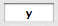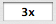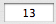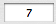﻿ Recognizing the patterns x^n and (-x)^n
RECOGNIZING THE PATTERNS $\,x^n\,$ and $\,(-x)^n$

EXAMPLES:

In the exercise, you will be filling in the blanks:

The expression $\,(-y)^{24}\,$ is of the form $\,(-x)^n\,$
where $\,x\,$ isand $\,n\,$ is.

Note that in the pattern $\,(-x)^n\,$,
the variable ‘$\,x\,$’ represents whatever comes after the minus sign,
and the variable ‘$\,n\,$’ represents the exponent.
The expression $\,(-3x)^{13}\,$ is of the form $\,(-x)^n\,$
where $\,x\,$ isand $\,n\,$ is.

Try not to be confused by the appearance of the variable ‘$\,x\,$’ in two places!
Again, we're matching something to the pattern $\,(-x)^n\,$,
where ‘$\,x\,$’ represents whatever comes after the minus sign.
What comes after the minus sign in $\,(-3x)^{13}\,$?
Answer: $\,3x\,$
The expression $\,(-2x)^{7}\,$ is of the form $\,x^n\,$
where $\,x\,$ isand $\,n\,$ is.

Here, we're matching something to the pattern $\,x^n\,$,
so ‘$\,x\,$’ represents the entire base.
Master the ideas from this section
Writing Expressions in the form $\,kx^n$
 The expression is of the form where $\,x\,$ is and $\,n\,$ is